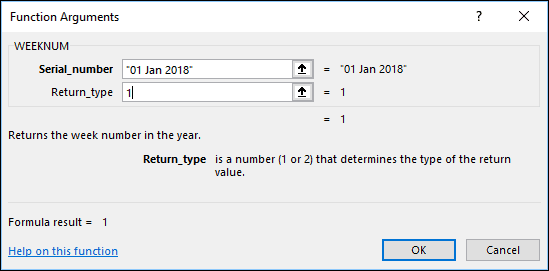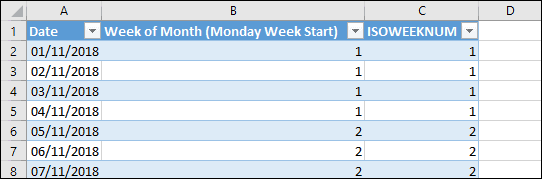# An Excel formula to get the week of month

It’s not unusual to want to split data into weeks. Excel recognizes this and provides the WEEKNUM function, which converts a date into a number between 1 and 52, representing which week of the year the date falls into.

If you’re unfamiliar with Excel formulas and functions, you could benefit greatly from our completely free Basic Skills e-book, which explains the basics of Excel formulas.

Sometimes you want to extract weeks within months instead of years. For example you want to identify which of your January sales fell into the first, second, third and fourth week of the month. This is much more of a challenge, as Excel doesn’t provide a built-in function that allows you to do this.

## How the WEEKNUM function works

It’s important to understand how WEEKNUM works before you can use it to extract the week of month from a date.

WEEKNUM accepts 2 arguments: a date and a rather cryptic Return_type.The Return_type controls which day the function will treat as the first day of the week. If you set this to 1 Excel will treat Sunday as the first day of the week. If you set it to 2 Excel will treat Monday as the first day of the week.

There are also 7 other options for different week starts. Clicking Help on this function will display a help file showing all of them. You can also see more about getting help in the free Basic Skills e-book.

## Extracting the week of the month

The formula to extract the week of month from a date is:

=WEEKNUM(A1,2)WEEKNUM(DATE(YEAR(A1),MONTH(A1),1),2)+1

The formula works as follows:

1. Extract the ‘week of year’ from the date.
2. Extract the ‘week of year’ from the date of the first day of the month the date falls within.
3. Subtract the two week numbers and add one (so that the first week does not appear as zero).

You can control which day is treated as the first day of the week by changing the second argument in both of the WEEKNUM functions:

=WEEKNUM(A1,2)-WEEKNUM(DATE(YEAR(A1),MONTH(A1),1),2)+1

The DATE, YEAR and MONTH functions are explained in depth in our Expert Skills Books and Ebooks.

## The ISOWEEKNUM function

ISOWEEKNUM is an alternative function that uses the ISO definition of week numbering. This treats Monday as the first day of the week and treats the first week of the year containing a Thursday as week number 1.

ISOWEEKNUM can be used instead of the WEEKNUM function:

=ISOWEEKNUM(A1)-ISOWEEKNUM(DATE(YEAR(A1),MONTH(A1),1))+1

## A working example### 6 thoughts on “An Excel formula to get the week of month”

1.Hi,

I need to know how to execute the below condition in excel through formulas mentioned in this forum-
E.g. 29th Oct 2018 to 30th Oct 2018 should fall in first week of November instead of Week 5 of October. In other words, my Week is starting on Saturday, So Oct 2018 will have 4 weeks only and date 29th Oct to 2nd Nov, 2018 should fall in Week 1 of November. The formula I am using as of now is as below –
=IF(K89=”No Date”,”No Week”,CONCAT(“Week “,WEEKNUM(I89-1)-WEEKNUM(I89-DAY(I89)-6)))

1.Hi Manoj,
The WEEKNUM function has an optional argument that lets you specify which day should be treated as the start of the week. To tell WEEKNUM to use Saturday as the first day of the week, use a formula like: =WEEKNUM(I89,16)

The ‘magic number’ 16 indicates that Saturday should be the start of the week, but you can see a list of all of the week start options in Excel’s help files.

2.So I have been trying to use the function to track two rolling week dates in excel, and the issue is that i cant get it to work consistently.

I am tracking Mondays, so using the 2 code.
The issue is that i need it to accurately roll from identifying the week span of wk5-wk1 to wk1-wk2 and so on. But because of how the week dates are spread apart if i set the spacing so that the mondays get tracked it will either show wk4-wk1 to wk1-wk2 or it will do wk5-wk1 to wk2-wk2.
Can you help me fix this?

1.Hi Jacob,
I’m afraid I’m having some trouble understanding your question, but it might help to know that there are two different algorithms that the WEEKNUM function can use. If you use 21 instead of 2 the function will use the alternate system of week numbers. You can find more information in the help file.

3.Hi, when I am calculating the week number of 09/27/2019, using =WEEKNUM(A1,2)-WEEKNUM(DATE(YEAR(A1),MONTH(A1),1),2)+1 then the result shows 5. But as per the Indian calendar, it should be 4. How can i get it?

1.Hi Abhishek,

The second parameter of the WEEKNUM function offers the choice of two different systems of week numbering. The formula shown above uses system 1, which treats the week containing the 1st of January as the first week of the year.
My guess is that the Indian calendar may use system 2, which treats the week containing the first Thursday of the year as the first week of the year.

You can easily modify the formula to use system 2 by changing it to:
=WEEKNUM(A1,21)-WEEKNUM(DATE(YEAR(A1),MONTH(A1),1),21)+1

Unfortunately this still seems to return a 5 for the date you mentioned, so my guess is that you may need one of the other options. There are a total of 10 different options that you can use to control how the week number is calculated and you can find them all listed on Microsoft’s help page for the WEEKNUM function.

Scroll to Top